Courses

# Test: Sheet Metal Operations - 2

## 30 Questions MCQ Test RRB JE for Mechanical Engineering | Test: Sheet Metal Operations - 2

Description
This mock test of Test: Sheet Metal Operations - 2 for Mechanical Engineering helps you for every Mechanical Engineering entrance exam. This contains 30 Multiple Choice Questions for Mechanical Engineering Test: Sheet Metal Operations - 2 (mcq) to study with solutions a complete question bank. The solved questions answers in this Test: Sheet Metal Operations - 2 quiz give you a good mix of easy questions and tough questions. Mechanical Engineering students definitely take this Test: Sheet Metal Operations - 2 exercise for a better result in the exam. You can find other Test: Sheet Metal Operations - 2 extra questions, long questions & short questions for Mechanical Engineering on EduRev as well by searching above.
QUESTION: 1

Solution:
QUESTION: 2

Solution:
QUESTION: 3

### The force requirement in a blanking operation of low carbon steel sheet is 5.0 kN. The thickness of the sheet is ‘t’ and diameter of the blanked part is ‘d’. For the same work material, ifthe diameter of the blanked part is increased to 1.5 d and thickness is reduced to 0.4 t, the new blanking force in kN is

Solution:
QUESTION: 4

In a blanking operation, the clearance is provided on

Solution:
QUESTION: 5

The cutting force in punching and blanking operations mainly depends on

Solution:
QUESTION: 6

Consider the following factors
1. Clearance between the punch and the die is too small.
2. The finish at the corners of the punch is poor.
3. The finish at the corners of the die is poor.
4. The punch and die alignment is not proper.

Q.

The factors responsible for the vertical lines parallel to the axis noticed on the outside of adrawn cylindrical cup would include.

Solution:
QUESTION: 7

In blanking operation, the clearance is provided on

Solution:
QUESTION: 8

Match List (Steel type) with List II (Product) and the correct answer using the codes given below the lists: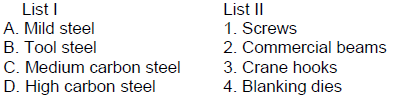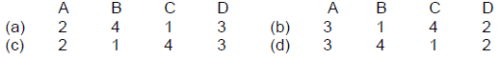Solution:
QUESTION: 9

For punching operation the clearance is provided on which one of the following?

Solution:

In punching useable part is punched sheet so size of hole must be accurate i.e.
size of punch must be accurate. Clearance have be given on Die only

QUESTION: 10

Assertion (A): A flywheel is attached to a punching press so as to reduce its speed fluctuations.
Reason(R): The flywheel stores energy: when its speed increase.

Solution:
QUESTION: 11

In drawing operation, proper lubrication is essential for which of the following reasons?
1. To improve die life
2. To reduce drawing forces
3. To reduce temperature
4. To improve surface finishSelect the correct answer using the code given below:

Solution:
QUESTION: 12

For 50% penetration of work material, a punch with single shear equal to thickness will

Solution:

When angle of shear = thickness, punch load increases from 0 to maximum at t/3,
and then remains same from t/3 to t, and reduce to zero at 4t/3.

QUESTION: 13

Match List I (Process) with List II (Production of parts) and select the correct answer using
the codes given below the lists: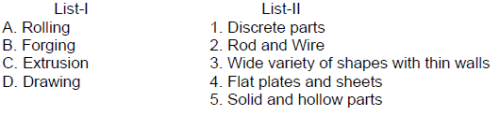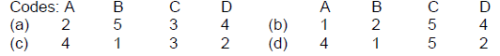Solution:
QUESTION: 14

Consider the following states of stress:
1. Compressive stress in flange
2. Tensile stress in the wall,
3. Tensile stress in the bottom part.

Q.

During drawing operation, the states of stress in cup would include

Solution:
QUESTION: 15

In deep drawing of sheets, the values of limiting draw ratio depends on

Solution:

The limit of deformation is reached when the load required deforming the flange becomes greater than the load-carrying capacity of the cup wall. Load carrying capacity of the wall = πDt x ft

QUESTION: 16

The tool for spinning copper is usually made for

Solution:

It is made from hard wood.

QUESTION: 17

For obtaining a cup of diameter 25 mm and height 15 mm by drawing, the size of the round blank should he approximately

Solution: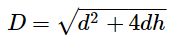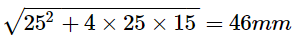QUESTION: 18

Which one of the following metals forming processes is not a high energy rate form ingprocess?

Solution:

High-Energy-Rate-Forming is metal forming through the application of large amount
of energy in a very sort time interval.
High energy-release rate can be obtained by five distinct methods:
(i) Underwater explosions.
(ii) Underwater spark discharge (electro-hydraulic).
(iii) Pneumatic-mechanical means.
(iv) Internal combustion of gaseous mixtures.
(v) Electro-magnetic (the use of rapidly formed magnetic fields)

QUESTION: 19

The process of removing the burrs or flash from a forged component in drop forging iscalled:

Solution:
QUESTION: 20

In the deep drawing of cups, blanks show a tendency to wrinkle up around the periphery(flange). The most likely cause and remedy of the phenomenon are, respectively,

Solution:
QUESTION: 21

Match List- I (Item to be produced) with List-II (Process to be used) and select the correct answer using the codes given below the lists: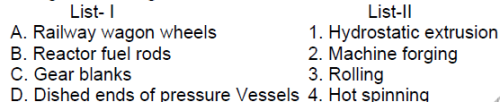Codes:

A    B   C     D                      A    B    C    D

(a)      2    1     3    4             (b)      3     1    2    4

(c)      4     3     1    2             (d)      3     4    1     2

Solution:
QUESTION: 22

Which one of the following is a high energy rate forming process?

Solution:
QUESTION: 23

Identify the stress - state in the FLANCE portion of a PARTIALLYDRAWN CYLINDRICALCUP when deep - drawing without a blank holder

Solution:
QUESTION: 24

Match List I (Operations) with List II (Stresses induced) and select the correct answer using the codes given below the Lists: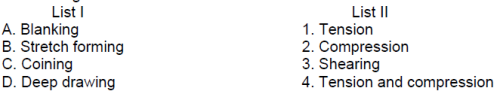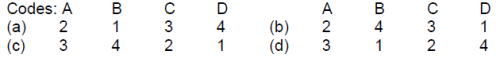Solution:
QUESTION: 25

Which one is not a method of reducing cutting forces to prevent the overloading of press?

Solution:
QUESTION: 26

A 2 mm thick metal sheet is to be bent at an angle of one radian with a bend radius of 100mm. If the stretch factor is 0.5, the bend allowance is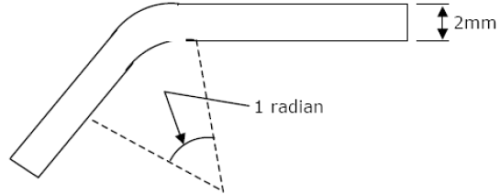Solution:
QUESTION: 27

The 'spring back' effect in press working is

Solution:
QUESTION: 28

Match the following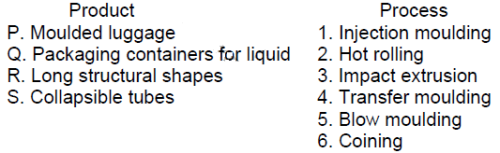Solution:
QUESTION: 29

Magnetic forming is an example of:

Solution:
QUESTION: 30

The thickness of the blank needed to produce, by power spinning a missile cone of thickness1.5 mm and half cone angle 30°, is

Solution: